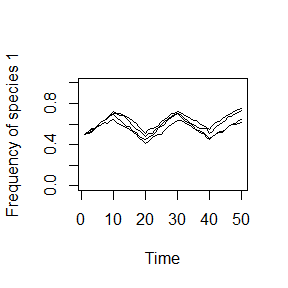## Online Box 3: R code to simulate temporally fluctuating selection: Figure 6.4

## specify the number of simulations, the number of years, and a matrix for output
num.sims <- 5 # number of independent simulations to run
num.years <- 50
freq.1.mat <- matrix(nrow = num.sims, ncol = num.years)

## start a loop for each of num.sims independent simulations
for (j in 1:num.sims) {

## specify parameters and initial conditions
J <- 4000
init.1 <- 0.5*J

COM <- vector(length = J)
COM[1:init.1] <- 1; COM[(init.1+1):J] <- 2
year <- 2

freq.1.mat[j,1] <- sum(COM==1)/J

# set fitness ratios in each sequence of 20 years
f20 <- c(rep(1.1,times=10),rep(1/1.1,times=10))
fit.ratios <- rep(f20,times=(1+num.years/20))

## run simulation
for (i in 1:(J*(num.years-1))) {

freq.1 <- sum(COM==1)/J; freq.2 <- 1 - freq.1
fit.ratio <- fit.ratios[year]
Pr.1 <- fit.ratio*freq.1/(fit.ratio*freq.1 + freq.2)
COM[ceiling(J*runif(1))] <- sample(c(1,2), 1, prob=c(Pr.1,1-Pr.1))

## record data
if (i %% J == 0) {
freq.1.mat[j,year] <- sum(COM==1)/J
year <- year + 1
}
}
}

## graph the results
plot(1:num.years, freq.1.mat[1,], type="l", xlab="Time",
ylab="Frequency of species 1", ylim=c(0,1))
for (i in 2:(num.sims-1)) {
lines(1:num.years,freq.1.mat[i,], type="l", ylim=c(0,1))
}### Description of the code. This code contains only a minor modification to the code in Online Box 2.

1. Instead of defining a single value of fit.ratio.avg (see Online Box 2), we define here a 20-year repeating sequence of average fitness ratios, f20. For 10 years, the fitness ratio is 1.1, and then 1/1.1 for the next 10 years (these values can be changed). The rep command repeats a value or vector a specified number of times in a new vector.
  f20 <- c(rep(1.1,times=10),rep(1/1.1,times=10))
fit.ratios <- rep(f20,times=(1+num.years/20))
1. Here we refer to the fit.ratios vector to define the value of fit.ratio in this year of the simulation. In the calculation of Pr.1, there is no term for freq.dep as this code includes no possible frequency dependence.
    fit.ratio <- fit.ratios[year]
Pr.1 <- fit.ratio*freq.1/(fit.ratio*freq.1 + freq.2)